# AP Physics C: Electricity and Magnetism Practice Test 10

### Test Information11 questions15 minutes

1. Which of these quantities decreases as the inverse square of distance for distances far from the objects producing the fields?

2. A proton enters a solenoid. Upon entry, the proton is moving in a straight line along the axis of the solenoid. Which of the following is a correct description of the proton’s motion within the solenoid?

3.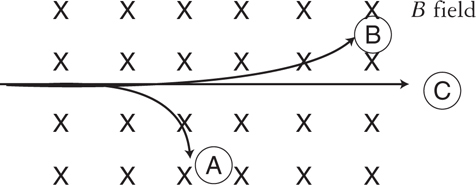A uniform magnetic field points into the page. Three subatomic particles are shot into the field from the left-hand side of the page. All have the same initial speed and direction. These particles take paths A, B, and C, as labeled in the diagram above. Which of the following is a possible identity for each particle?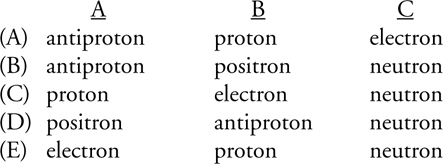A     B     C

4.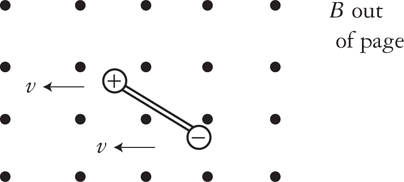The electric dipole shown above consists of equal-magnitude charges and has an initial leftward velocity v in a uniform magnetic field pointing out of the page, as shown above. The dipole experiences

5. A beam of electrons has speed 107 m/s. It is desired to use the magnetic field of the Earth, 5 × 10−5 T, to bend the electron beam into a circle. What will be the radius of this circle?

6.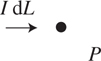A very small element of wire of length dL carries a current I. What is the direction of the magnetic field produced by this current element at point P, shown above?

7.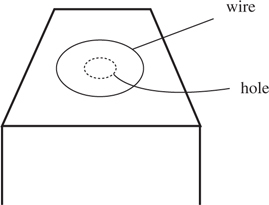A loop of wire surrounds a hole in a table, as shown above. A bar magnet is dropped, north end down, from far above the table through the hole. Let the positive direction of current be defined as counterclockwise as viewed from above. Which of the following graphs best represents the induced current I in the loop?

8.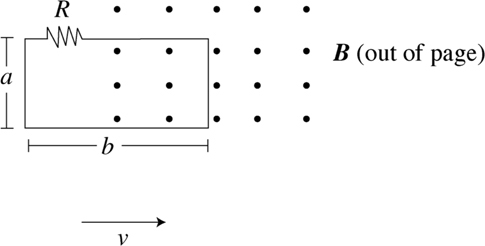A rectangular loop of wire has dimensions a × b and includes a resistor R. This loop is pulled with speed v from a region of no magnetic field into a uniform magnetic field B pointing through the loop, as shown above. What is the magnitude and direction of the current through the resistor?

9.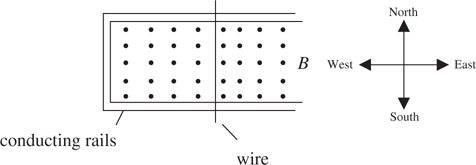A conducting wire sits on smooth metal rails, as shown above. A variable magnetic field points out of the page. The strength of this magnetic field is increased linearly from zero. Immediately after the field starts to increase, what will be the direction of the current in the wire and the direction of the wire’s motion?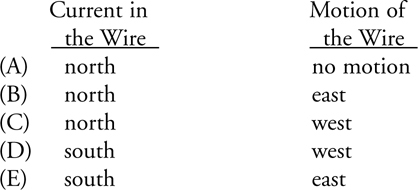Current in the Wire     Motion of the Wire

10.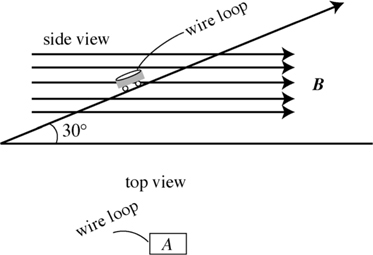A uniform magnetic field B points parallel to the ground. A toy car is sliding down a frictionless plane inclined at 30°. A loop of wire of resistance R and cross-sectional area A lies in the flat plane of the car’s body, as shown above. What is the magnetic flux through the wire loop?

11.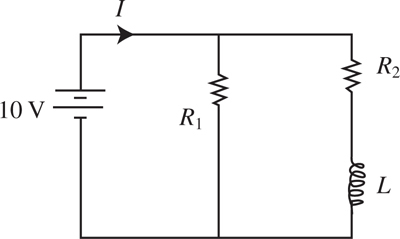If the two equal resistors R1 and R2 are connected in parallel to a 10-V battery with no other circuit components, the current provided by the battery is I. In the circuit shown above, an inductor of inductance L is included in series with R2. What is the current through R2 after the circuit has been connected for a long time?